# Intermediate Geometry : How to find the percentage of a sector from an angle

## Example Questions

← Previous 1

### Example Question #1 : How To Find The Percentage Of A Sector From An Angle

The central angle of a sector is 72o. What percentage of the circle is comprised by the sector?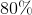Explanation:

The arc length of a sector, in degrees, is equal to the central angle. The total number of a degrees of a circle is 360. Therefore, we can use a proportion to calculate the percentage.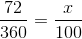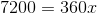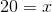Therefore, 20% of the circle is comprised of the sector.

### Example Question #2 : How To Find The Percentage Of A Sector From An Angle

A sector of a circle has a central angle of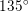.  What percentage of the circle does the sector occupy?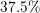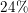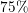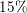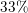Explanation:

A whole circle is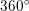.  We therefore need to find the percent.  We can do this by dividing.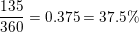### Example Question #3 : How To Find The Percentage Of A Sector From An Angle

A survey was given to 250 high schoolers asking them how they got to school each day.  The sector representing the students that ride the bus is 100 degrees, the sector representing the students that drive their own car is 90 degrees, and the sector representing the students that walk or ride their bike is 85 degrees.  What percent of students get to school in another manner (parents drop them off, etc)?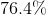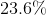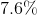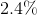Explanation:

To find the missing percentage, first you can add the 3 sectors' angle measures together to get 275 degrees.

Then subtract that from 360 degrees because you want this missing sector, which is 85 degrees.

Then set-up a proportion: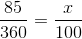and solve.

### Example Question #4 : How To Find The Percentage Of A Sector From An Angle

A circle graph is used to depict the results from a survey given to high school students regarding their favorite sport. The sector representing baseball measures 52 degrees.  What percent of the high schoolers who took the survey prefer baseball over any other sport?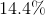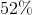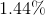Explanation:

To convert the angle of a sector in a circle to the percentage of that sector you can set-up a proportion: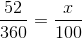and then solve.  The total number of degrees in a circle is 360, so you place 52 out of 360. The total percent being represented by a circle is 100, so you place(what you are trying to find) out of 100.

### Example Question #5 : How To Find The Percentage Of A Sector From An Angle

A circle graph represents the different types of pie you can find at a local bakery. The blueberry pie wedge measures 120 degrees, the apple pie wedge measures 110 degrees and the cherry pie wedge measures 130 degrees.  What percent of pies in the bakery are either blueberry or cherry?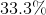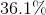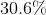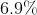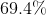Explanation:

First add together the degrees that represent blueberry and cherry pie to get 250 degrees.  You want to include both sectors because the question is asking for the percent of pies that is either blueberry or cherry.

Then set up a proportion: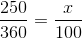and solve for.

### Example Question #6 : How To Find The Percentage Of A Sector From An Angle

A circle graph represents the student population at a local high school.  The sector representing boys measures 200 degrees.  What percent of the high school is girls?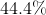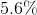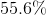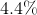Explanation:

First subtract 200 from 360 to get the number of degrees making up the sector representing girls.

Then set-up a proportion: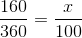and solve for.

### Example Question #7 : How To Find The Percentage Of A Sector From An Angle

A survey was given to 300 local townspeople regarding their favorite local dish. The results of the survey are below, with the number of degrees of each sector representing each dish following.

Fried chicken, 87 degrees

Steak and potatoes, 75 degrees

Corned beef hash, 82 degrees

Bison burger, 22 degrees

What percent of the local townspeople prefer a dish that includes chicken?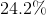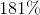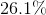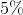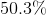Explanation:

First add together the number of degrees of both sectors representing a dish with chicken (fried chicken and chicken enchiladas), 87 + 94.

Then set-up a proportion with this sum: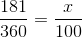and solve for.

### Example Question #8 : How To Find The Percentage Of A Sector From An Angle

A pie chart represents the ages of children in a local basketball league.  The data is shown below, with the age range of each sector followed by the number of degrees of that sector.

Ages 2-4, 20 degrees

Ages 5-7, 30 degrees

Ages 8-10, 100 degrees

Ages 11-13, 90 degrees

Ages 14-16, ? degrees

What percent of the children in the basketball league are between the ages of 14-16?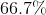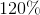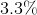Explanation:

First add together the degrees of the sectors representing children ages 2-13. Then take that sum, 240 degrees, away from the total degrees of the circle, 360 degrees.

Finally set-up a proportion with the remaining degrees (120) which represents the sector of children ages 14-16.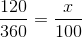.

### Example Question #9 : How To Find The Percentage Of A Sector From An Angle

A circle chart represents the survey results of the following question:

How many hours a week do you typically spend watching TV?

The results are below, with the sector title and the number of degrees of each sector following.

Less than 1 hour, 40 degrees

1-4 hours, 45 degrees

5-8 hours, 30 degrees

9-12 hours, 100 degrees

13-16 hours, 85 degrees

17 hours or more, 60 degrees

What percent of people surveyed watch TV for 9 or more hours a week?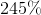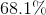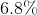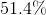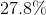Explanation:

First, add together the degrees from the 3 sectors that include 9 or more hours of TV: 100 degrees, 85 degrees and 60 degrees.

Take this sum, 245 degrees, and set-up a proportion: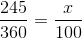and solve for.

### Example Question #10 : How To Find The Percentage Of A Sector From An Angle

A circle graph represents the demographics of a major US city.  The data is shown below with the category followed by the number of degrees making up that category's sector.

Black or African-American: 100 degrees

Hispanic or Latino: 100 degrees

White: 80 degrees

Two or more races: ? degrees

What percent of the city's population classifies themselves as being 2 or more races?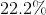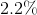Explanation:

First, add together the degrees of the known sectors: 100 degrees, 100 degrees, and 80 degrees.

Subtract this sum (280 degrees) from the total number of degrees in a circle (360 degrees).

Take this difference to set-up a proportion:and solve for.

← Previous 1